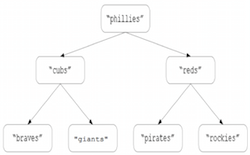### CSC 421: Algorithm Design and Analysis Spring 2019 HW3: Divide/Decrease & Conquer Trees

In CSC 321, we considered basic implementations of binary trees and binary search trees. The following classes are variations of the versions from last semester: TreeNode.java, BinaryTree.java, BinarySearchTree.java. The `BinaryTree` class contains several methods that use a divide-and-conquer approach, including an O(N) `size` method. The `BinarySearchTree` class, which inherits from `BinaryTree`, overrides the `add` and `contains` methods with O(log N) decrease-and-conquer versions. It also contains inefficient O(N) implementations of `select` and `rank`, which you will similarly replace with O(log N) decrease-and-conquer versions.

### Part 1: O(N) `asList`

Currently, the `asList` method is incomplete. Implement this method using a divide-and-conquer approach. In particular, start with an empty list and then recursively traverse the tree (using an inorder traversal), adding each data item to the list. Finally, return that list. Note that this method is used by the `toString` method, so it should be possible to print the contents of a binary tree after you have completed the method.

### Part 2: O(1) `size`

Currently, the `size` method uses divide-and-conquer recursion to traverse the tree and count the number of nodes. To avoid the O(N) cost of this approach, you are to modify `TreeNode` so that in addition to storing the data value and subtrees, each node also stores the sizes of its subtrees (and those values are updated when either subtree is changed). With this size information embedded in each node, it becomes simple to determine the size of any subtree in a tree. For example, suppose `current` referred to a node in a binary tree. if you knew that the left subtree of `current` contained 12 nodes, and the right subtree of `current` contained 14 nodes, then the tree rooted at `current` would contain 12+14+1 = 27 nodes.

Note: all of the `TreeNode` methods should remain O(1).

Once you have made your modifications, reimplement the `size` method in `BinaryTree` so that it utilizes the size information in nodes to determine the tree size in O(1) time.

### Part 3: O(log N) `select`

Currently, the `select` method in `BinarySearchTree` is O(N), since it first converts the tree into a list (using your `asList` method) and then accesses the corresponding index in that list. Alternatively, a decrease-and-conquer approach can be taken, similar to the approach used in the quick-select algorithm described in lectures. Consider the following binary search tree:Suppose you want the rank-4 value in the tree. Since there are 3 values in the left subtree, the rank-4 value must be at the root. If you wanted the rank-3 value in the tree, it must be in the left subtree (and in fact is the rank-3 value in the left subtree). If you wanted the rank-5 value in the tree, it must be in the right subtree (and in fact is the rank-1 value in the right subtree).

You are to reimplement the `select` operation using this decrease-and-conquer approach, which does work proportional to the height of the binary search tree. As long as the tree is relatively balanced, the `select` operation will be O(log N).

### Part 4: O(log N) `rank`

Similarly, it is possible to utilize the size information in nodes to implement an O(log N) `rank` operation. The `rank` operation is the inverse of `select` - it finds a specific value in the tree and returns its order rank. For example, the rank of "cubs" in the above binary search tree is 2, whereas the rank of "pirates" is 5. If the `rank` method is called on a value that does not appear in the tree, it should return -1.

You are to reimplement the `rank` method using a decrease-and-conquer approach, so that the amount of work is proportional to the height of the binary search tree.